Share

# RD Sharma solutions for Class 8 Maths chapter 15 - Understanding Shapes-I (Polygons) [Latest edition]

Course
Textbook page

#### Chapters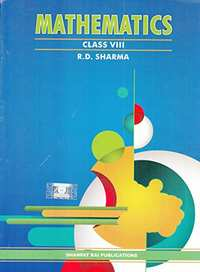## Chapter 15: Understanding Shapes-I (Polygons)

Ex. 15.1

#### RD Sharma solutions for Class 8 Maths Chapter 15 Understanding Shapes-I (Polygons) Exercise 15.1 [Pages 5 - 6]

Ex. 15.1 | Q 1.1 | Page 5

Draw rough diagram to illustrate the following  Open curve .

Ex. 15.1 | Q 1.2 | Page 5

Draw rough diagram to illustrate the following  Closed curve .

Ex. 15.1 | Q 2.1 | Page 5

Classify the following curve as open or closed: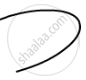Ex. 15.1 | Q 2.2 | Page 5

Classify the following curve as open or closed: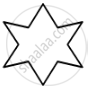Ex. 15.1 | Q 2.3 | Page 5

Classify the following curve as open or closed: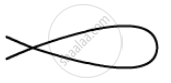Ex. 15.1 | Q 2.4 | Page 5

Classify the following curve as open or closed: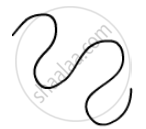Ex. 15.1 | Q 2.5 | Page 5

Classify the following curve as open or closed: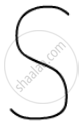Ex. 15.1 | Q 2.6 | Page 5

Classify the following curve as open or closed: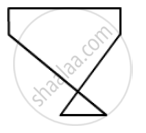Ex. 15.1 | Q 3 | Page 5

Draw a polygon and shade its interior. Also draw its diagonals, if any.

Ex. 15.1 | Q 4.1 | Page 6

Illustrate, if posible, one of the following with a rough diagram:

A colsed curve that is not a polygon.

Ex. 15.1 | Q 4.2 | Page 6

Illustrate, if posible, one of the following with a rough diagram:

An open curve made up entirely of line segments.

Ex. 15.1 | Q 4.3 | Page 6

Illustrate, if posible, one of the following with a rough diagram:

A polygon with two sides.

Ex. 15.1 | Q 5 | Page 6

Following are some figures: Classify each of these fugures on the basis of the following:
(i) Simple curve
(ii) Simple closed curve
(iii) Polygon
(iv) Convex polygon
(v) Concave polygon
(vi) Not a curve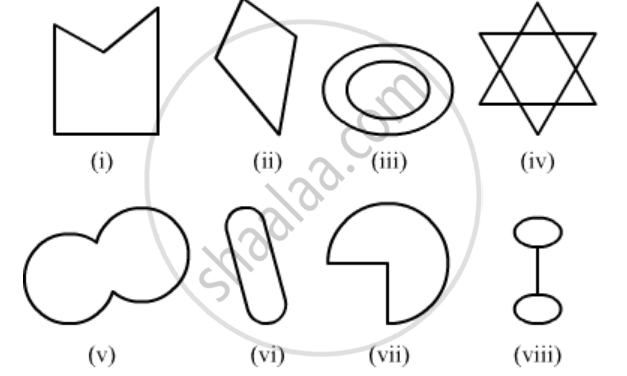Ex. 15.1 | Q 6.1 | Page 6

How many diagonal does of the following have A convex quadrilateral .

Ex. 15.1 | Q 6.2 | Page 6

How many diagonal does of the following have A regular hexagon .

Ex. 15.1 | Q 6.3 | Page 6

How many diagonal does of the following have A triangle .

Ex. 15.1 | Q 7.1 | Page 6

What is a regular polygon? State the name of a regular polygon of 3 sides .

Ex. 15.1 | Q 7.2 | Page 6

What is a regular polygon? State the name of a regular polygon of  4 sides .

Ex. 15.1 | Q 7.3 | Page 6

What is a regular polygon? State the name of a regular polygon of 6 sides .

## Chapter 15: Understanding Shapes-I (Polygons)

Ex. 15.1## RD Sharma solutions for Class 8 Maths chapter 15 - Understanding Shapes-I (Polygons)

RD Sharma solutions for Class 8 Maths chapter 15 (Understanding Shapes-I (Polygons)) include all questions with solution and detail explanation. This will clear students doubts about any question and improve application skills while preparing for board exams. The detailed, step-by-step solutions will help you understand the concepts better and clear your confusions, if any. Shaalaa.com has the CBSE Class 8 Maths solutions in a manner that help students grasp basic concepts better and faster.

Further, we at Shaalaa.com provide such solutions so that students can prepare for written exams. RD Sharma textbook solutions can be a core help for self-study and acts as a perfect self-help guidance for students.

Concepts covered in Class 8 Maths chapter 15 Understanding Shapes-I (Polygons) are Regular and Irregular Polygons, Angle Sum Property, Sum of the Measures of the Exterior Angles of a Polygon, Concept of Trapezium, Concept of Kite, Elements of a Parallelogram, Diagonals of a Parallelogram, Concept of Rhombus, Concept of a Rectangle, Classification of Polygons, Concept of Diagonals, Convex and Concave Polygons, Introduction of Quadrilaterals, Concept of a Square, Concept of Parallelogram.

Using RD Sharma Class 8 solutions Understanding Shapes-I (Polygons) exercise by students are an easy way to prepare for the exams, as they involve solutions arranged chapter-wise also page wise. The questions involved in RD Sharma Solutions are important questions that can be asked in the final exam. Maximum students of CBSE Class 8 prefer RD Sharma Textbook Solutions to score more in exam.

Get the free view of chapter 15 Understanding Shapes-I (Polygons) Class 8 extra questions for Class 8 Maths and can use Shaalaa.com to keep it handy for your exam preparation